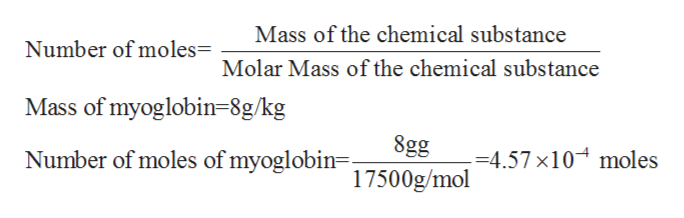# The molecular weight of sperm whale myoglobin is 17.8 kDa.17.8 kDa. The myoglobin content of sperm whale muscle is about 80 g · kg−1.80 g · kg−1. In contrast, the myoglobin content of some human muscles is about 8 g · kg−1.8 g · kg−1.Compare the amounts of O2O2 bound to myoglobin in human muscle and in sperm whale muscle. Assume that the myoglobin is saturated with O2,O2, and that the molecular weights of human and sperm whale myoglobin are the same.How much O2O2 is bound to myoglobin in human muscle?How much O2O2 is bound to myoglobin in whale muscle?The amount of oxygen dissolved in tissue water at 37°C37°C is about 3.5×10−5 M.3.5×10−5 M. What is the ratio of myoglobin‑bound oxygen to dissolved oxygen in the tissue water of sperm whale muscle?

Question
372 views

The molecular weight of sperm whale myoglobin is 17.8 kDa.17.8 kDa. The myoglobin content of sperm whale muscle is about 80 g · kg−1.80 g · kg−1. In contrast, the myoglobin content of some human muscles is about 8 g · kg−1.8 g · kg−1.

Compare the amounts of O2O2 bound to myoglobin in human muscle and in sperm whale muscle. Assume that the myoglobin is saturated with O2,O2, and that the molecular weights of human and sperm whale myoglobin are the same.

How much O2O2 is bound to myoglobin in human muscle?

How much O2O2 is bound to myoglobin in whale muscle?

The amount of oxygen dissolved in tissue water at 37°C37°C is about 3.5×10−5 M.3.5×10−5 M. What is the ratio of myoglobin‑bound oxygen to dissolved oxygen in the tissue water of sperm whale muscle?

check_circle

Step 1

Molar mass of a given chemical compound is calculated by the addition of standard atomic masses of the atoms forming the given compound.

Myoglobin refers to an iron and oxygen binding protein which is found in the muscle tissues of vertebrates and is found almost in all the mammals.

Step 2

The molar mass of myoglobin is given to be 17.5 kDa which is equals to 17500g/mol.

It is given that the myoglobin content of some human muscles is around 8 g/kg.

Therefore, the number of moles of myoglobin found in the human muscles can be calculated as follows:help_outlineImage TranscriptioncloseMass of the chemical substance Number of moles= Molar Mass of the chemical substance Mass of myoglobin=8g/kg| 8gg Number of moles of myoglobin= 1500g/mol =4.57 x10 moles 17 fullscreen
Step 3

Now the myoglobin is known to bind to one oxygen molecule per molecu...

### Want to see the full answer?

See Solution

#### Want to see this answer and more?

Solutions are written by subject experts who are available 24/7. Questions are typically answered within 1 hour.*

See Solution
*Response times may vary by subject and question.
Tagged in

### Chemistry